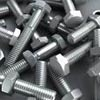You may also likeChess

What would be the smallest number of moves needed to move a Knight from a chess set from one corner to the opposite corner of a 99 by 99 square board?2001 Spatial Oddity

With one cut a piece of card 16 cm by 9 cm can be made into two pieces which can be rearranged to form a square 12 cm by 12 cm. Explain how this can be done.Screwed-up

A cylindrical helix is just a spiral on a cylinder, like an ordinary spring or the thread on a bolt. If I turn a left-handed helix over (top to bottom) does it become a right handed helix?

Matching Frieze Patterns

Age 11 to 14 Challenge Level:

The simplest patterns are 3 and 12 in which the motif is repeated by a translation along the strip. Translations are the only transformations involved. (p p p p p p p)

In patterns 1 and 5 the motif is rotated and then the motif (with this copy) is repeated by a translation. This pattern involves half turns and translations. (p d p d p d p d)

In patterns 7 and 13 there is a reflection in the horizontal axis and then the motif (with this copy) is repeated by a translation. This pattern involves reflections in the horizontal axis and translations. (E E E E E E)

In patterns 4 and 10 the first copy is made by a reflection in the horizontal axis combined with a translation (a glide reflection) and then the motif (with this copy) is repeated by a translation. This pattern involves glide reflections in the horizontal axis and translations. (p b p b p b p b p b)

In patterns 9 and 11 the first copy is made by a reflection in a mirror line perpendicular to the strip and then the motif (with this copy) is repeated by a translation. This pattern involves reflections in a perpendicular axis and translations. (b d b d b d b d b d)

In patterns 6 and 14 the motif is repeated by a combination of two reflections in the horizontal and perpendicular axes and then the motif (with this copy) is repeated by a translation. This pattern involves reflections in the horizontal and in a perpendicular axis, half turns and translations. (H H H H H H H H)

In patterns 2 and 8 the motif is repeated by a combination of a reflections in a perpendicular axis and a glide reflection and then the motif (with this copy) is repeated by a translation. This pattern involves reflections in a perpendicular axis, glide reflections, half turns and translations. (pqbd pqbd pqbd pqbd)